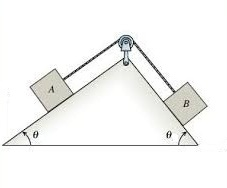# Mechanics - 5Find the sum of the magnitudes of the acceleration of the blocks $a \text{ m/s}^2$, tension in the string $T \text{ N}$, and frictional force acting on $B$ by the inclined surface $f_B \text{ N}$. Give your answer to the nearest integer.

Assumptions and Details:

• No friction between the inclined surface and block $A$
• The coefficient of friction between the inclined surface and block $B$ is $\mu_B = 0.2$
• The string is massless and inextensible.
• The pulley is massless and there is no friction in the pulley and with the string.
• Mass of block $A$, $m_A = \text{3 kg}$
• Mass of block $B$, $m_B = \text{5 kg}$
• Angle of incline, $\theta = \sin^{-1} \dfrac35$
• Acceleration due to gravity, $g = \text{10 m/s}^2$

Try my World of Physics to solve many problems like this one.

×